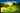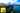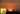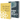# Logistic Regression with Gradient Descent in JavaScript

by Robin WieruchA couple of my recent articles gave an by solving with linear regression using gradient descent or normal equation.

In a regression problem, an algorithm is trained to predict continuous values. It can be housing prices in a specific area based on a feature set such as square meters or numbers of bedrooms. The algorithm is trained by using a training set. Afterward, the algorithm can predict housing prices for houses not included in the training set. Checkout the recent articles to understand the foundational knowledge about linear regression including the essential cost function and hypothesis to perform the gradient descent algorithm. This article doesn't recap those topics but applies them for logistic regression to solve a classification problem in JavaScript.

In contrast, in a classification problem an algorithm is trained to predict categorical values. For instance, a classification problem could be to separate spam emails from useful emails or to classify transactions into fraudulent and not fraudulent. The output would be a binary dependent variable, because it can be either 0 or 1. However, a classification problem can be extended to a multiclass classification problem going beyond the binary classification. For instance, a set of articles could be classified into different topics such as web development, machine learning or software engineering.

An algorithm that could be used to solve a classification problem is called logistic regression. In the following article, I want to guide you through the theory and implementation of logistic regression with gradient descent in JavaScript.

I highly recommend to take the Machine Learning course by Andrew Ng. This article will not explain the machine learning algorithms in detail, but only demonstrate their usage in JavaScript. The course on the other hand goes into detail and explains these algorithms in an amazing quality. At this point in time of writing the article, I learn about the topic myself and try to internalize my learnings by writing about them and applying them in JavaScript. If you find any parts for improvements, please reach out in the comments or create a Issue/Pull Request on GitHub.

## The Decision Boundary

In a logistic regression, the training set is classified by a hypothesis function to put each data point into a group of labels. If it is a binary classification, the training set can be classified into positive and negative labels. For instance, in a training set for fraud detection in bank transactions, it is already known whether a transaction is marked as positive or negative of being fraudulent. That way the algorithm can be trained on an existing training set. In the following example, the training set has a feature size of 2 (x1, x2) and classifies the labels (y) into two groups.

`x1 +   |               0   |   |                    0              0   |                               0   |   |                1           0   |   |     1   |   |       1         1   |                           1   |       1   |   +---------------------------------------+                                          x2`

Starting with an initial hypothesis function, the theta parameters of the hypothesis can be trained to draw a line that classifies the data points into two groups. The trained hypothesis could be as simple as a linear function using a straight line to classify the groups.

`x1 +   |               0   |XXX   |   XXX              0              0   |      XXX                      0   |         XXX   |            XXX 1           0   |               XXX   |     1            XXX   |                     XXX   |       1         1      XXX   |                           1XX   |       1                      XXX   |                                  XXX   +---------------------------------------+                                          x2`

However, the hypothesis can be way more complex. If a training set cannot be separated by a straight line, the hypothesis function can be a . For instance, in the following training set the hypothesis function would need to describe something similar to a circle instead of a straight line.

`x1 +   |               0   |                       0       0   |   0                 1   |            1                   1    0   |    0                    1   |                1           1         0   |   |     0     1        1            1   |                            1          0   |       0         1   |                           1   |       0          0   |            0                   0   +---------------------------------------+                                          x2`

The line defined by the hypothesis function that classifies the data points is called decision boundary. As you have seen by now, the decision boundary can be linear or non-linear.

## Logistic Regression Model (Hypothesis)

In logistic regression, same as for linear regression, a hypothesis function with its parameters theta is trained to predict future values. The polynomial order of the hypothesis function stays fixed from the beginning, but its parameters change over the training phase. The hypothesis function can be as simple as a linear function for a two feature training set.

`h(x) => thetaZero + thetaOne * x1 + thetaTwo * x2`

When using the , it boils down to a of theta and the training set.

`h(x) => theta' * X`

However, in a classification problem, the output of the hypothesis function should be either 0 or 1. It should either classify that a data point belongs to a group or doesn't belong to it. That's why the hypothesis function cannot be used as in a linear regression problem, because in linear regression the output isn't between 0 and 1. The hypothesis function needs to output the probability of x being a positive y.

`0 <= h(x) <= 1`

That's where the sigmoid function (logistic function) function comes into play.

`           1g(z) => --------              -z         1 + e`

It classifies an input z to either being in the group positive or negative labels. If z is high, the output is close to 1. If z is low, the output is close to 0.

`                     1 +                       |             XXXXXX                       |        XXXXX                       |      XX                       |    XX                       |  XX                       | X                       |X                   0.5 X                      X|                     X |                   XX  |                 XX    |              XX       |         XXXXX         |   XXXXXX            0 |   +-------------------+-------------------+                                           z`

Now functional composition comes in handy because you can pass the hypothesis function from linear regression into the sigmoid function. The output is the hypothesis function for logistic regression.

`h(x) => g(theta' * X)`

Substituting z would lead to the following equation.

`           1h(x) => --------              -theta' * X         1 + e`

That's the final logistic regression model to train the theta parameters. It uses theta parameters and the training set X (without the output labels y) to output values between 0 and 1 (y).

## Logistic Regression and the Cost Function

Before gradient descent can be used to train the hypothesis in logistic regression, the cost functions needs to be defined. It is needed to compute the cost for a hypothesis with its parameters regarding a training set. By using gradient descent, the cost should decrease over time. However, the cost function is not the same as in a regression problem, because it would lead to a lot of local optima when applying it to the sigmoid function in a classification problem. The function would describe a non-convex graph and thus lead gradient descent to different non optimal minima.

`   +   |   |XX                                           XX   |  XX                                       XX   |    XX  XX                           XX  XX   |      XX  XX                       XX  XX   |            XX  XX           XX  XX   |              XX  XX       XX  XX   |                    XX   XX   |                       XX   +----------------------------------------------+`

Instead, in order to have a more effective approach finding the minimum, the cost function has to describe a convex graph.

`   +   |   |XX                                                   XX   |  XX                                               XX   |    XX                                           XX   |      XX                                       XX   |        XXX                                 XXX   |           XXX                           XXX   |              XXX                     XXX   |                 XXXX             XXXX   |                     XXXX     XXXX   |                         XXXXX   +-------------------------------------------------------+`

That way, the derivative of the cost function can be used to make gradient steps towards the minimum without being stuck in any non optimal minimum. Thus, in a classification problem using logistic regression, a logarithmic function is applied to compute the costs for both positive and negative values.

`                 -log(h(x))      if y = 1cost(h(x), y) =>                 -log(1 - h(x))  if y = 0`

If you would plot those functions, you could see how the cost increases or decreases for input x and output y. Now, instead of using two equations, it can be simplified to one equation when y is always 0 or 1. The article leaves out the process of deriving the simplified version.

`cost(h(x), y) => -y * log(h(x)) - (1 - y) * log(1 - h(x))`

Using the simplified version, it becomes more of a straight forward approach to apply the cost function in a programmatic way later on. Keep in mind, that this cost function only applies to one data point in the training set. The final cost function will return the sum of the costs from all data points in the training set divided by the size of the training set. The goal of gradient descent is to minimize the cost.

## Cost Function in Logistic Regression with JavaScript

So far, everything mentioned was theoretical. Now let's apply these learnings in JavaScript by implementing the cost function in JavaScript for logistic regression.

Imagine a training set about students which has the result of two exams and a binary variable if the student was admitted for university. The training set has the size m (m = 100, each row a student) with features n (n = 2, exam result one, exam result two). It can be expressed in a matrix. Furthermore, the label y (y = 1 if student is admitted for university) can be expressed in a matrix too.

Now imagine a function in JavaScript that has access to the training set in its function signature. You can split up the training set into input matrix X and output vector y.

`function init(matrix) {   // Part 0: Preparation  console.log('Part 0: Preparation ...\n');   let X = math.eval('matrix[:, 1:2]', {    matrix,  });  let y = math.eval('matrix[:, 3]', {    matrix,  });   ...}`

Let's see how those matrices could look like for a small training set of m = 5.

`console.log(matrix); // [//   [34.62365962451697, 78.0246928153624, 0],//   [30.28671076822607, 43.89499752400101, 0],//   [35.84740876993872, 72.90219802708364, 0],//   [60.18259938620976, 86.30855209546826, 1],//   [79.0327360507101, 75.3443764369103, 1],// ] console.log(X); // [//   [34.62365962451697, 78.0246928153624],//   [30.28671076822607, 43.89499752400101],//   [35.84740876993872, 72.90219802708364],//   [60.18259938620976, 86.30855209546826],//   [79.0327360507101, 75.3443764369103],// ] console.log(y); // [//   ,//   ,//   ,//   ,//   ,// ]`

Just by looking at the inputs and outputs, you could guess that a higher exam score could lead to a higher chance of being admitted at university.

Let's get back to the implementation. As little helper for later on, you can retrieve the dimensions of the training set and the feature set.

`function init(matrix) {   // Part 0: Preparation  console.log('Part 0: Preparation ...\n');   let X = math.eval('matrix[:, 1:2]', {    matrix,  });  let y = math.eval('matrix[:, 3]', {    matrix,  });   let m = y.length;  let n = X.length;   ...}`

Now, let's lay out the framework for the cost function. The cost function will be called with the fixed input and output matrices. Also the theta parameters will be used in the cost function.

`function init(matrix) {   // Part 0: Preparation  console.log('Part 0: Preparation ...\n');   let X = math.eval('matrix[:, 1:2]', {    matrix,  });  let y = math.eval('matrix[:, 3]', {    matrix,  });   let m = y.length;  let n = X.length;   // Part 1: Cost Function and Gradient   ...   let cost = costFunction(theta, X, y);   ...} function costFunction(theta, X, y) {  ...   return cost;}`

But the theta parameters are not defined yet. Since we are using a vectorized implementation, theta needs to be a vector with the size of the features n + 1. In the following, theta will be populated as vector with zeros.

`function init(matrix) {   ...   // Part 1: Cost Function and Gradient   let theta = Array(n + 1).fill().map(() => );  let cost = costFunction(theta, X, y);   ...}`

Later on, the theta parameters of the hypothesis function will be trained by using gradient descent. That's where the cost function can be used to verify that the cost decreases over time for specific vectors of theta. In the beginning, we can use the cost function simply to output the cost for a arbitrary vector of theta (e.g. [, , ]).

One step is missing, before implementing the cost function. The input matrix X needs to add an intercept term. Only that way the matrix operations work for the dimensions of theta and matrix X.

`function init(matrix) {   ...   // Part 1: Cost Function and Gradient   // Add Intercept Term  X = math.concat(math.ones([m, 1]).valueOf(), X);   let theta = Array(n + 1).fill().map(() => );  let cost = costFunction(theta, X, y);   ...}`

Now let's implement the cost function. Basically you can split it up into two equations - one for the hypothesis function and one for the cost function. The equation that we are going to use for the cost function in logistic regression was mentioned in the theoretical part of the article before.

`function costFunction(theta, X, y) {   const m = y.length;   let h = math.eval(`X * theta`, {    X,    theta,  });   const cost = math.eval(`(1 / m) * (-y' * log(h) - (1 - y)' * log(1 - h))`, {    h,    y,    m,  });   return cost;}`

So far, the cost function is only applicable for a regression problem, but not for a classification problem with logistic regression. The sigmoid function is missing which is composed around the hypothesis function.

`function sigmoid(z) {  ...   return g;} function costFunction(theta, X, y) {   const m = y.length;   let h = sigmoid(math.eval(`X * theta`, {    X,    theta,  }));   const cost = math.eval(`(1 / m) * (-y' * log(h) - (1 - y)' * log(1 - h))`, {    h,    y,    m,  });   return cost;}`

The equation for the sigmoid function was mentioned before too.

`function sigmoid(z) {  let g = math.eval(`1 ./ (1 + e.^-z)`, {    z,  });   return g;}`

That's it. Last but not least, you can output the cost for the untrained theta parameters.

`function init(matrix) {   ...   // Part 1: Cost Function and Gradient   // Add Intercept Term  X = math.concat(math.ones([m, 1]).valueOf(), X);   let theta = Array(n + 1).fill().map(() => );  let cost = costFunction(theta, X, y);   console.log('cost: ', cost);  console.log('\n');}`

Essentially you can come up with any theta vector on your own to reduce the cost manually, but we will use gradient descent in the next part of the article to train the hypothesis with its theta parameters.

## Logistic Regression with Gradient Descent in JavaScript

Gradient descent is the essential part to train the theta parameters of the hypothesis function. It is an iterative process which adjusts the parameters by reducing the cost over time. The equation for gradient descent is defined as:

`repeat {                                  d  theta  => theta  - alpha * ----------- * J(theta)       j                      d * theta                                       j}`

Basically, each theta parameter is derived from the previous theta paramater subtracted by the learning rate times the derivative term of the cost function. You might recall that it is the same equation as for a regression problem. But when you substitute J with the cost function and the hypothesis in the cost function with the hypothesis for logistic regression (which includes the sigmoid function), you get a different equation for gradient descent in logistic regression than for linear regression.

Let's get to the implementation of gradient descent for logistic regression in JavaScript. First, define the necessary constants such as learning rate alpha, number of iterations and an initial vector for the theta parameter which will be trained eventually.

`function init(matrix) {   // Part 0: Preparation   ...   // Part 1: Cost Function and Gradient   ...   // Part 2: Gradient Descent   const ALPHA = 0.001;  const ITERATIONS = 500;   theta = [[-25], , ];  theta = gradientDescent(X, y, theta, ALPHA, ITERATIONS);} function gradientDescent(X, y, theta, ALPHA, ITERATIONS) {  ...   return theta;}`

Because gradient descent can be initialized with arbitrary theta, it is up to you to choose the values for theta. Depending on the initial theta parameters, gradient descent can end up in different local minimum. It must not be the global minimum. The theta example from the code snippet come pretty close to minimizing the cost though.

Last but not least, the gradient descent for the logistic regression needs to be implemented. First, it iterates over the given number of iterations to train theta in the loop.

`function gradientDescent(X, y, theta, ALPHA, ITERATIONS) {  const m = y.length;   for (let i = 0; i < ITERATIONS; i++) {    ...  }   return theta;}`

Second, it trains theta based on the training set, the learning rate, the previous theta parameters and the hypothesis.

`function gradientDescent(X, y, theta, ALPHA, ITERATIONS) {  const m = y.length;   for (let i = 0; i < ITERATIONS; i++) {    ...     theta = math.eval(`theta - ALPHA / m * ((h - y)' * X)'`, {      theta,      ALPHA,      m,      X,      y,      h,    });  }   return theta;}`

Third, the hypothesis function is missing. By using the sigmoid function to compose the hypothesis function, we can solve the classification problem with gradient descent.

`function gradientDescent(X, y, theta, ALPHA, ITERATIONS) {  const m = y.length;   for (let i = 0; i < ITERATIONS; i++) {    let h = sigmoid(math.eval(`X * theta`, {      X,      theta,    }));     theta = math.eval(`theta - ALPHA / m * ((h - y)' * X)'`, {      theta,      ALPHA,      m,      X,      y,      h,    });  }   return theta;}`

That's it. Your theta parameters and thus your hypothesis should be trained over the defined number of iterations with the learning rate alpha.

Last but not least, you can output your trained theta parameters and calculate the cost for it. It should be lower than for any hand picked theta parameters.

`function init(matrix) {   // Part 0: Preparation   ...   // Part 1: Cost Function and Gradient   ...   // Part 2: Gradient Descent (without feature scaling)   const ALPHA = 0.001;  const ITERATIONS = 400;   theta = [[-25], , ];  theta = gradientDescent(X, y, theta, ALPHA, ITERATIONS);   cost = costFunction(theta, X, y)   console.log('theta: ', theta);  console.log('\n');  console.log('cost: ', cost);  console.log('\n');}`

Finally, you can predict new input data points, a student with two exams, by using your trained hypothesis function. It should output a probability whether a student is admitted at university.

`function init(matrix) {   // Part 0: Preparation   ...   // Part 1: Cost Function and Gradient   ...   // Part 2: Gradient Descent   ...   // Part 3: Predict admission of a student with exam scores 45 and 85   let studentVector = [1, 45, 85];  let prob = sigmoid(math.eval('studentVector * theta', {    studentVector,    theta,  }));   console.log('Predicted admission for student with scores 45 and 85 in exams: ', prob);}`

You can find an example of the logistic regression with gradient descent in JavaScript in one of my GitHub repositories. If you like it, make sure to star it :-)

## Multiclass Classification in Logistic Regression

So far, the article described binary classification problems whereas the output should be either positive or negative. But what about a multiclass classification? For instance, tagging emails for different domains such as work, friends and family could be done by using a multiclass classification with logistic regression. Thus imagine you would want to classify your data into those 3 categories. Therefore the training set could be visualized as in the following.

`x1 +   |               C   |   |                    C              C   |                               C   |   |                C           C   |   |     A   |   |  A     A         B               B   |                           B   B   |       A   |   +---------------------------------------+                                          x2`

Now, it seems impossible to draw a decision boundary for 3 categories. That's why in a multiclass classification a one-vs-all classification (one-vs-rest classification) is used for logistic regression. In the case of the A, B and C classes, it could be A vs the rest.

`x1 +   |               0   |   |                    0              0   |                               0   |   |                0           0   |   |     1   |   |  1     1         0               0   |                           0   0   |       1   |   +---------------------------------------+                                          x2`

Since all the classes were substituted to two classes, the decision boundary can be drawn between the one class and the remaining classes.

`x1 +   |               0   |   |XXX                 0              0   |   XXX                         0   |      XXX   |         XXX    0           0   |            XXX   |     1         XXX   |                  XXX   |  1     1         0  XXX          0   |                        XXX0   0   |       1                   XXX   |                              XXX   +---------------------------------------+                                          x2`

The approach is taken for every class and thus there would be 3 decision boundaries in the end. The classifier h(x) for each category gets trained to predict the probability y of x being in a category. When the hypotheses are trained eventually, a new data point can be classified by picking the class that maximizes the probability.

There is no implementation in JavaScript for a multiclass classification with logistic regression yet. Perhaps thats your chance to contribute to the organization! Reach out to me if you want to start a repository as sample project for other machine learning in JavaScript beginners.

In conclusion, I hope the walkthrough was useful for you to understand logistic regression and using it with gradient descent in JavaScript. If you are sharing the article, it would make me aware of people actually wanting to read more about those topics. I learn the topic myself, so please leave a comment if I can apply any improvements to the article.## Linear Regression with Normal Equation in JavaScript

A recent article gave an introduction to the field of machine learning in JavaScript by predicting housing prices with gradient descent in a univariate regression problem. It used plain mathematical…## Improving Gradient Descent in JavaScript

A recent article gave an introduction to the field of machine learning in JavaScript by predicting housing prices with gradient descent in a univariate regression problem. There are several…